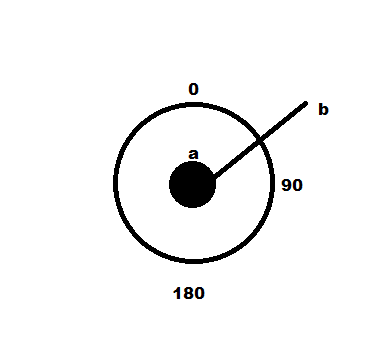# Find rotation from 2 points

I’ve been looking around and don’t think I’ve found what I’ve been looking for.

I’m trying to get a rotation from 2 2d points.

According to the picture below, what can I use to find the rotation of the two points?

For example, from the info given below I would get somewhere around 45 oOne approach is to use Mathf.Atan2(). Given two vector3 v3A and v3B, your code would be something like:

``````var v3 = v3B - v3A;
var angle = Mathf.Atan2(v3.y, v3.x);
``````

Note that if you are looking towards positive ‘Z’, the angle returned by Atan2() will have ‘0’ where you have the ‘90’ label, and the angle will wrap the opposite direction. If the exact angles you have a above are important, you will have to make the conversion.

A second way to approach this problem would be to calculate the signed angle between two vectors. Assuming the diagram is looking at the XY plane, you could get the angles in the form you define by using Vector3.up as the reference and the vector AB as the second vector. If you searched for “Unity3d signed angle between two vectors,” you will find many hits including this one: